# COST-VOLUME-PROFIT RELATIONSHIPS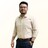Student at Daffodil International University - DIU um Daffodil International University - DIU
24. Aug 2016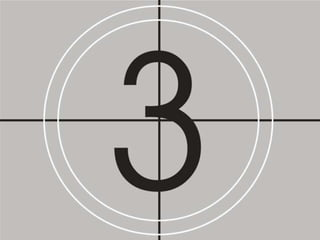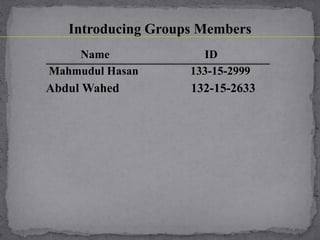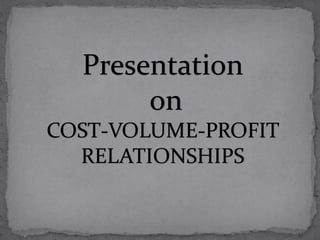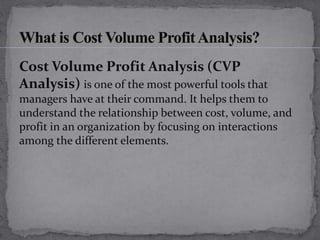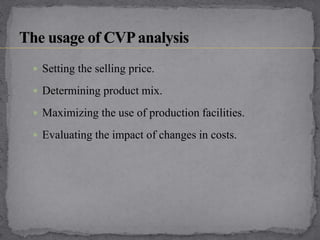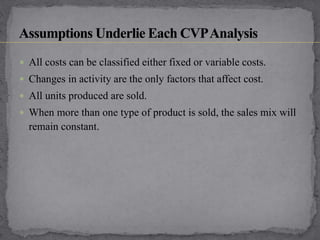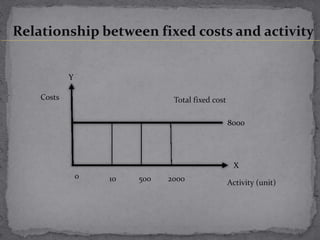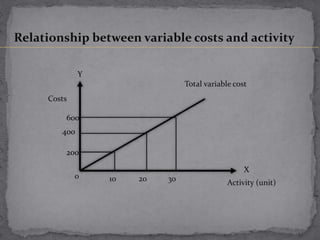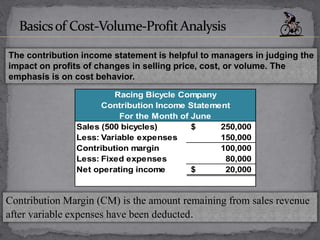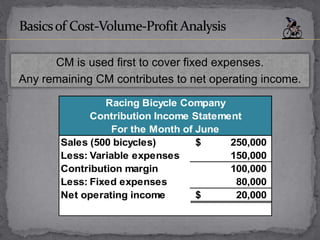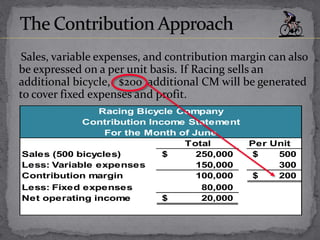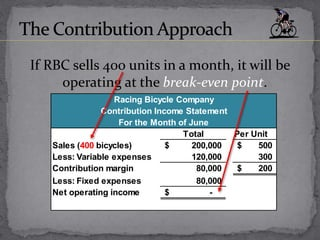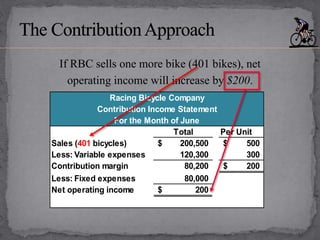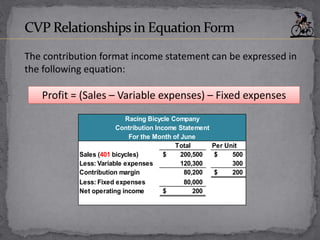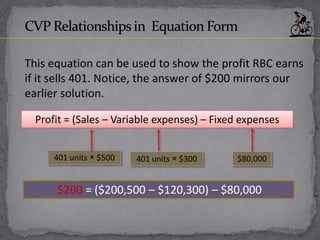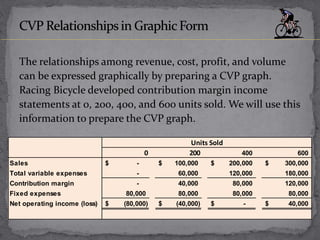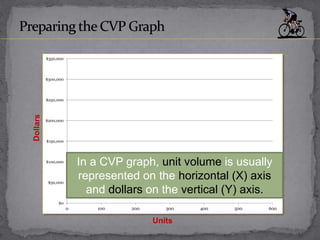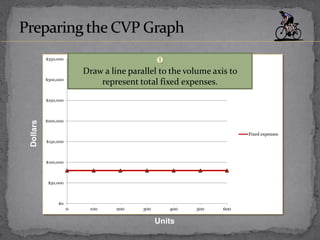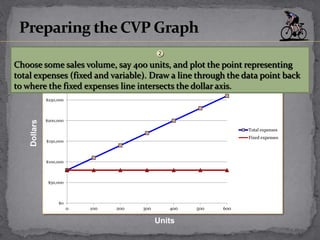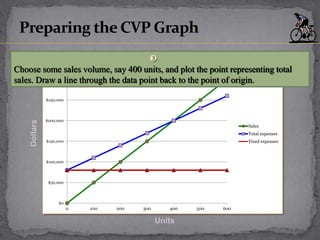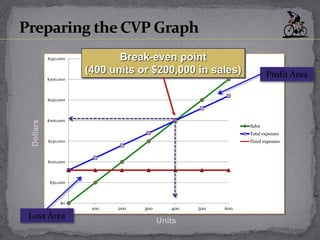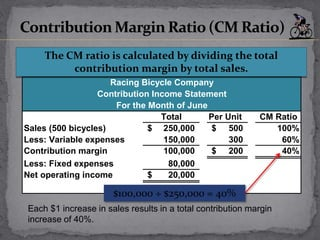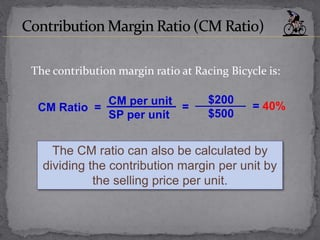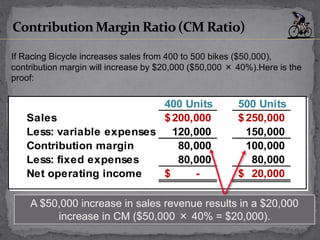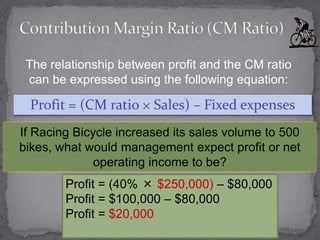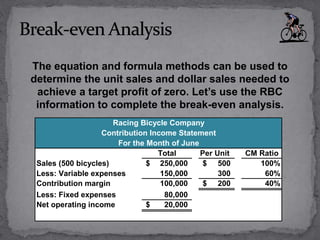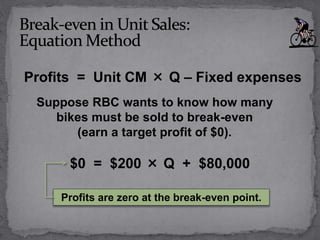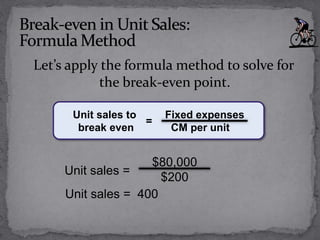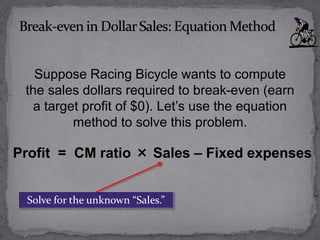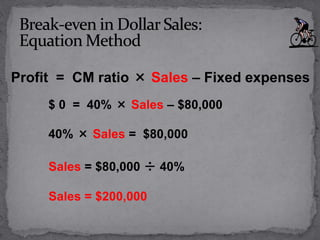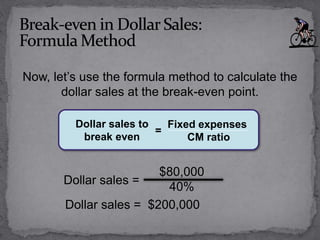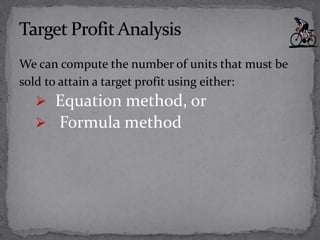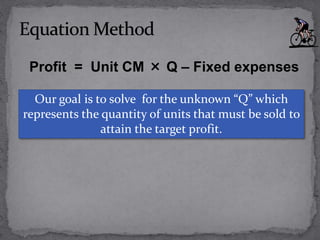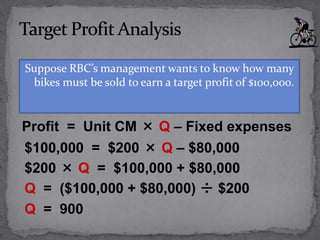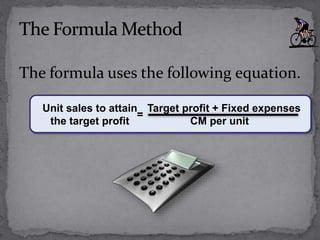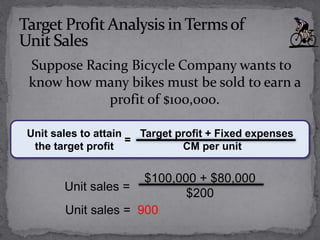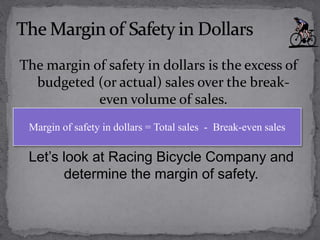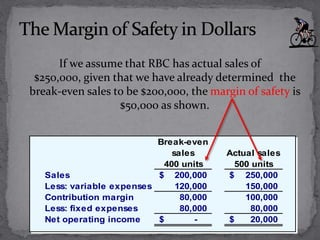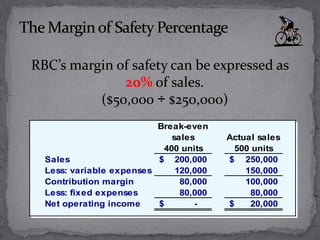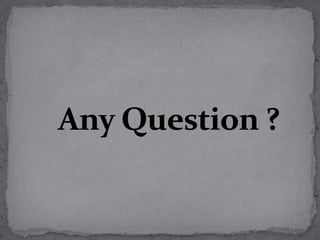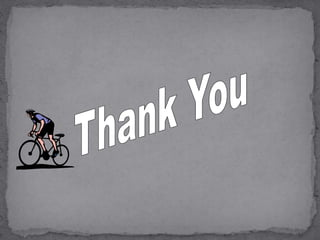1 von 44

### COST-VOLUME-PROFIT RELATIONSHIPS

• 5. Name ID Mahmudul Hasan 133-15-2999 Abdul Wahed 132-15-2633 Introducing Groups Members
• 6. Presentation on COST-VOLUME-PROFIT RELATIONSHIPS
• 7. Cost Volume Profit Analysis (CVP Analysis) is one of the most powerful tools that managers have at their command. It helps them to understand the relationship between cost, volume, and profit in an organization by focusing on interactions among the different elements.
• 8.  Setting the selling price.  Determining product mix.  Maximizing the use of production facilities.  Evaluating the impact of changes in costs.
• 9.  All costs can be classified either fixed or variable costs.  Changes in activity are the only factors that affect cost.  All units produced are sold.  When more than one type of product is sold, the sales mix will remain constant.
• 10. Relationship between fixed costs and activity Costs Activity (unit) Total fixed cost 10 500 20000 8000 Y X
• 11. Relationship between variable costs and activity Costs Activity (unit) Total variable cost 10 20 300 200 400 600 Y X
• 12. Contribution Margin (CM) is the amount remaining from sales revenue after variable expenses have been deducted. Sales (500 bicycles) 250,000\$ Less: Variable expenses 150,000 Contribution margin 100,000 Less: Fixed expenses 80,000 Net operating income 20,000\$ Racing Bicycle Company Contribution Income Statement For the Month of June The contribution income statement is helpful to managers in judging the impact on profits of changes in selling price, cost, or volume. The emphasis is on cost behavior.
• 13. CM is used first to cover fixed expenses. Any remaining CM contributes to net operating income. Sales (500 bicycles) 250,000\$ Less: Variable expenses 150,000 Contribution margin 100,000 Less: Fixed expenses 80,000 Net operating income 20,000\$ Racing Bicycle Company Contribution Income Statement For the Month of June
• 14. Total Per Unit Sales (500 bicycles) 250,000\$ 500\$ Less: Variable expenses 150,000 300 Contribution margin 100,000 200\$ Less: Fixed expenses 80,000 Net operating income 20,000\$ Racing Bicycle Company Contribution Income Statement For the Month of June Sales, variable expenses, and contribution margin can also be expressed on a per unit basis. If Racing sells an additional bicycle, \$200 additional CM will be generated to cover fixed expenses and profit.
• 15. Total Per Unit Sales (400 bicycles) 200,000\$ 500\$ Less: Variable expenses 120,000 300 Contribution margin 80,000 200\$ Less: Fixed expenses 80,000 Net operating income -\$ Racing Bicycle Company Contribution Income Statement For the Month of June If RBC sells 400 units in a month, it will be operating at the break-even point.
• 16. Total Per Unit Sales (401 bicycles) 200,500\$ 500\$ Less: Variable expenses 120,300 300 Contribution margin 80,200 200\$ Less: Fixed expenses 80,000 Net operating income 200\$ Racing Bicycle Company Contribution Income Statement For the Month of June If RBC sells one more bike (401 bikes), net operating income will increase by \$200.
• 17. The contribution format income statement can be expressed in the following equation: Profit = (Sales – Variable expenses) – Fixed expenses Total Per Unit Sales (401 bicycles) 200,500\$ 500\$ Less: Variable expenses 120,300 300 Contribution margin 80,200 200\$ Less: Fixed expenses 80,000 Net operating income 200\$ Racing Bicycle Company Contribution Income Statement For the Month of June
• 18. This equation can be used to show the profit RBC earns if it sells 401. Notice, the answer of \$200 mirrors our earlier solution. 401 units × \$500 401 units × \$300 \$80,000 Profit = (Sales – Variable expenses) – Fixed expenses \$200 = (\$200,500 – \$120,300) – \$80,000
• 19. The relationships among revenue, cost, profit, and volume can be expressed graphically by preparing a CVP graph. Racing Bicycle developed contribution margin income statements at 0, 200, 400, and 600 units sold. We will use this information to prepare the CVP graph. 0 200 400 600 Sales -\$ 100,000\$ 200,000\$ 300,000\$ Total variable expenses - 60,000 120,000 180,000 Contribution margin - 40,000 80,000 120,000 Fixed expenses 80,000 80,000 80,000 80,000 Net operating income (loss) (80,000)\$ (40,000)\$ -\$ 40,000\$ Units Sold
• 20. \$0 \$50,000 \$100,000 \$150,000 \$200,000 \$250,000 \$300,000 \$350,000 0 100 200 300 400 500 600 Units In a CVP graph, unit volume is usually represented on the horizontal (X) axis and dollars on the vertical (Y) axis.
• 21. \$0 \$50,000 \$100,000 \$150,000 \$200,000 \$250,000 \$300,000 \$350,000 0 100 200 300 400 500 600 Fixed expenses Units  Draw a line parallel to the volume axis to represent total fixed expenses.
• 22. \$0 \$50,000 \$100,000 \$150,000 \$200,000 \$250,000 \$300,000 \$350,000 0 100 200 300 400 500 600 Total expenses Fixed expenses Units  Choose some sales volume, say 400 units, and plot the point representing total expenses (fixed and variable). Draw a line through the data point back to where the fixed expenses line intersects the dollar axis.
• 23. \$0 \$50,000 \$100,000 \$150,000 \$200,000 \$250,000 \$300,000 \$350,000 0 100 200 300 400 500 600 Sales Total expenses Fixed expenses Units  Choose some sales volume, say 400 units, and plot the point representing total sales. Draw a line through the data point back to the point of origin.
• 24. \$0 \$50,000 \$100,000 \$150,000 \$200,000 \$250,000 \$300,000 \$350,000 0 100 200 300 400 500 600 Sales Total expenses Fixed expenses Break-even point (400 units or \$200,000 in sales) Units Loss Area Profit Area
• 25. Total Per Unit CM Ratio Sales (500 bicycles) 250,000\$ 500\$ 100% Less: Variable expenses 150,000 300 60% Contribution margin 100,000 200\$ 40% Less: Fixed expenses 80,000 Net operating income 20,000\$ Racing Bicycle Company Contribution Income Statement For the Month of June \$100,000 ÷ \$250,000 = 40% The CM ratio is calculated by dividing the total contribution margin by total sales. Each \$1 increase in sales results in a total contribution margin increase of 40%.
• 26. The contribution margin ratio at Racing Bicycle is: The CM ratio can also be calculated by dividing the contribution margin per unit by the selling price per unit. CM per unit SP per unit CM Ratio = = 40% \$200 \$500 =
• 27. 400 Units 500 Units Sales 200,000\$ 250,000\$ Less: variable expenses 120,000 150,000 Contribution margin 80,000 100,000 Less: fixed expenses 80,000 80,000 Net operating income -\$ 20,000\$ A \$50,000 increase in sales revenue results in a \$20,000 increase in CM (\$50,000 × 40% = \$20,000). If Racing Bicycle increases sales from 400 to 500 bikes (\$50,000), contribution margin will increase by \$20,000 (\$50,000 × 40%).Here is the proof:
• 28. The relationship between profit and the CM ratio can be expressed using the following equation: Profit = (CM ratio × Sales) – Fixed expenses Profit = (40% × \$250,000) – \$80,000 Profit = \$100,000 – \$80,000 Profit = \$20,000 If Racing Bicycle increased its sales volume to 500 bikes, what would management expect profit or net operating income to be?
• 29. The equation and formula methods can be used to determine the unit sales and dollar sales needed to achieve a target profit of zero. Let’s use the RBC information to complete the break-even analysis. Total Per Unit CM Ratio Sales (500 bicycles) 250,000\$ 500\$ 100% Less: Variable expenses 150,000 300 60% Contribution margin 100,000 200\$ 40% Less: Fixed expenses 80,000 Net operating income 20,000\$ Racing Bicycle Company Contribution Income Statement For the Month of June
• 30. \$0 = \$200 × Q + \$80,000 Profits = Unit CM × Q – Fixed expenses Suppose RBC wants to know how many bikes must be sold to break-even (earn a target profit of \$0). Profits are zero at the break-even point.
• 31. Let’s apply the formula method to solve for the break-even point. Unit sales = 400 \$80,000 \$200 Unit sales = Fixed expenses CM per unit = Unit sales to break even
• 32. Suppose Racing Bicycle wants to compute the sales dollars required to break-even (earn a target profit of \$0). Let’s use the equation method to solve this problem. Profit = CM ratio × Sales – Fixed expenses Solve for the unknown “Sales.”
• 33. Profit = CM ratio × Sales – Fixed expenses \$ 0 = 40% × Sales – \$80,000 40% × Sales = \$80,000 Sales = \$80,000 ÷ 40% Sales = \$200,000
• 34. Now, let’s use the formula method to calculate the dollar sales at the break-even point. Dollar sales = \$200,000 \$80,000 40% Dollar sales = Fixed expenses CM ratio = Dollar sales to break even
• 35. We can compute the number of units that must be sold to attain a target profit using either:  Equation method, or  Formula method
• 36. Profit = Unit CM × Q – Fixed expenses Our goal is to solve for the unknown “Q” which represents the quantity of units that must be sold to attain the target profit.
• 37. Suppose RBC’s management wants to know how many bikes must be sold to earn a target profit of \$100,000. Profit = Unit CM × Q – Fixed expenses \$100,000 = \$200 × Q – \$80,000 \$200 × Q = \$100,000 + \$80,000 Q = (\$100,000 + \$80,000) ÷ \$200 Q = 900
• 38. The formula uses the following equation. Target profit + Fixed expenses CM per unit = Unit sales to attain the target profit
• 39. Suppose Racing Bicycle Company wants to know how many bikes must be sold to earn a profit of \$100,000. Target profit + Fixed expenses CM per unit = Unit sales to attain the target profit Unit sales = 900 \$100,000 + \$80,000 \$200 Unit sales =
• 40. The margin of safety in dollars is the excess of budgeted (or actual) sales over the break- even volume of sales. Margin of safety in dollars = Total sales - Break-even sales Let’s look at Racing Bicycle Company and determine the margin of safety.
• 41. If we assume that RBC has actual sales of \$250,000, given that we have already determined the break-even sales to be \$200,000, the margin of safety is \$50,000 as shown. Break-even sales 400 units Actual sales 500 units Sales 200,000\$ 250,000\$ Less: variable expenses 120,000 150,000 Contribution margin 80,000 100,000 Less: fixed expenses 80,000 80,000 Net operating income -\$ 20,000\$
• 42. RBC’s margin of safety can be expressed as 20% of sales. (\$50,000 ÷ \$250,000) Break-even sales 400 units Actual sales 500 units Sales 200,000\$ 250,000\$ Less: variable expenses 120,000 150,000 Contribution margin 80,000 100,000 Less: fixed expenses 80,000 80,000 Net operating income -\$ 20,000\$